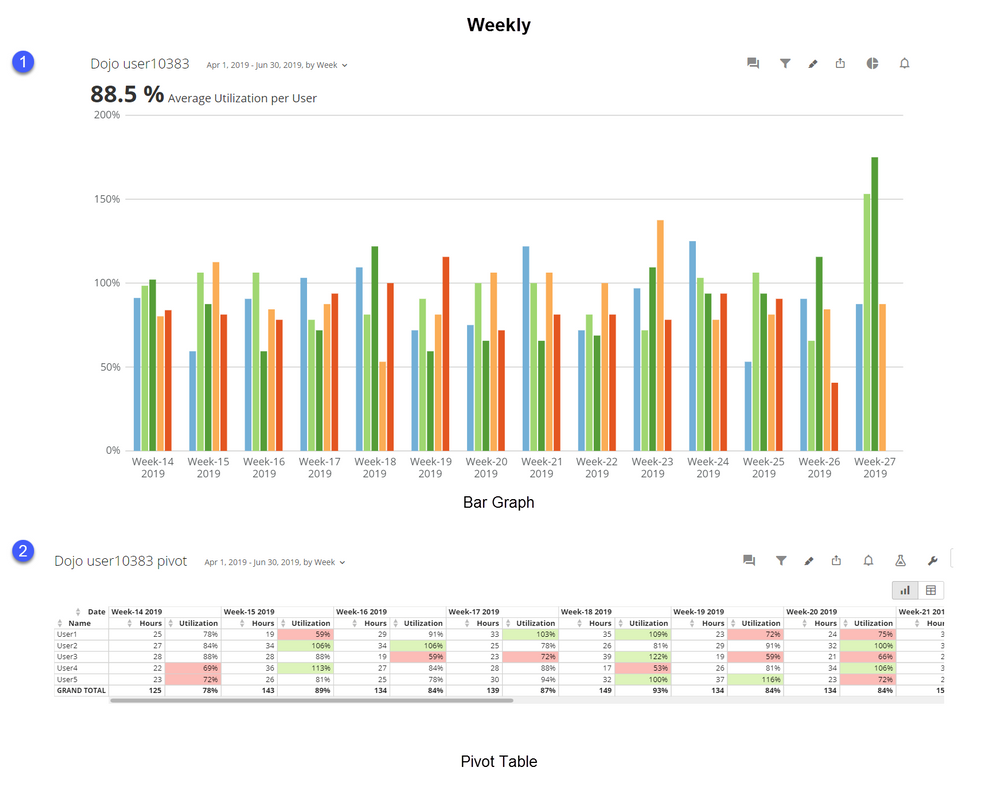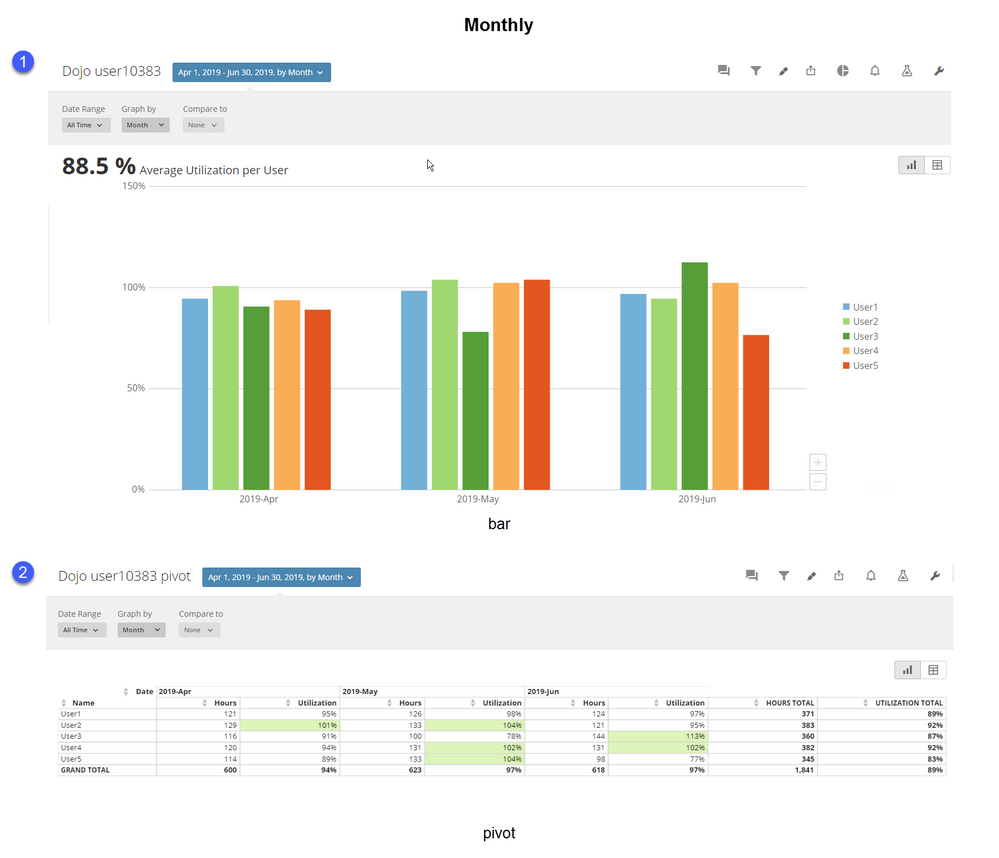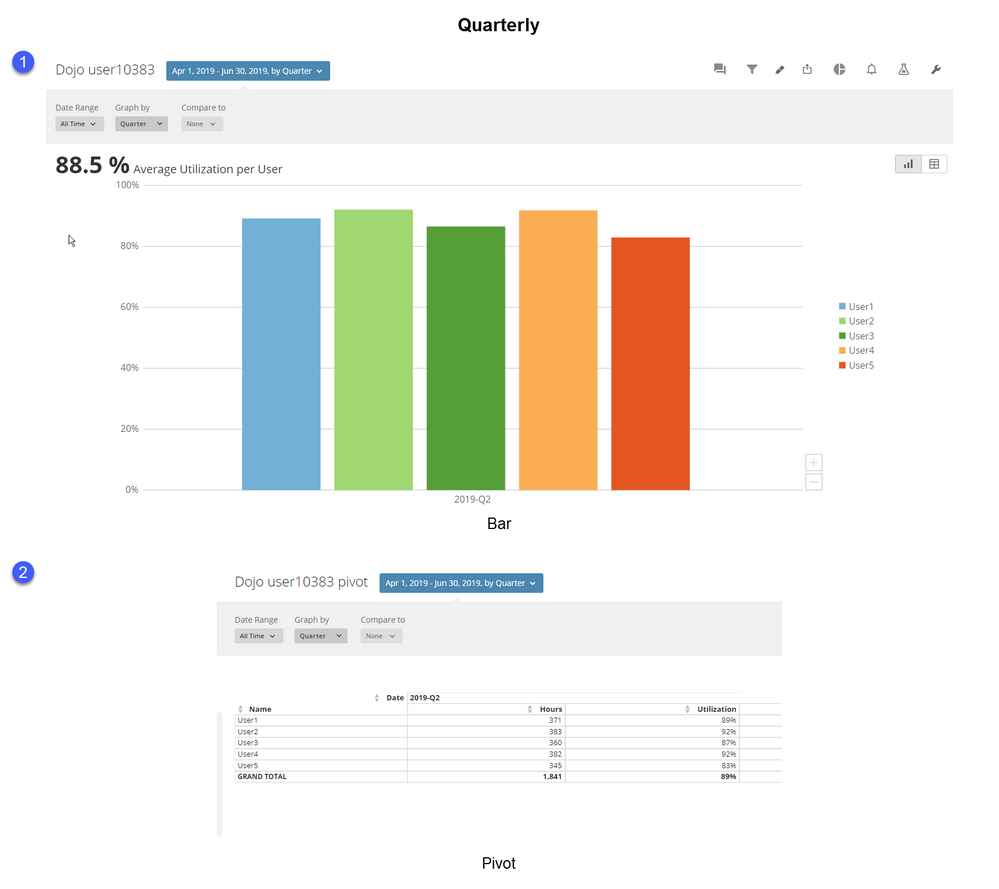# Utilization Calculation

Hello Team,

I'm trying to calculation utilization that has the ability to roll up or down. Currently, our team is based on a 32 hour work week. I have a measure in my data set that sum our hours entered by day. I was able to get the caculation to work using this logic SUM(`Hours`/32) but I need the abilitiy or create a calculated field that aggregates 32 by week, month, quarter, year. So a workers weekly target hours is 32 week, 128 month and 416 quarter. Any idea can to create this caculated fields or beast mode?

• How many days are in your work week?

Assuming 5 days, then you could calculate utilization using something like this:

`sum(`Hours`) / (32 * CEILING(COUNT(DISTINCT `Date`) / 5))`

This would look at the number of dates that a user has entered hours for and divide it by 5.  If they logged more than 5 days, say 7 days... then it would use 64 as the denominator because it would expect that you were looking at a 2 week period.  If they logged hours for 15 to 20 days, then it would be looking for 128 hours.  Between 60 and 65 days would be a quarter, or 416 hours for 100% utilization.

Hope that helps.

______________________________________________________________________________________________
“There is a superhero in all of us, we just need the courage to put on the cape.” -Superman
______________________________________________________________________________________________
• Thank you for the help but it's still not working. I've attached a screenshot. The new utilzation is your calcualtion and utilization is mine that works at the week level.

• OK.  So this is what I did.  I'm not sure what your dataset looks like, but I assume you have a Date, Employee (either ID or name), and a field for hours worked each day.

I made a dataset with dates from 4/1/2019 to 6/30/2019 (Q2) and populated it with 5 users and a random number between 0 and 8 for each day.

Then I used this formula for utilization:

`(sum(`Hours`) /(CASEwhen COUNT(DISTINCT `Date`) <= 7 then (32*(count(distinct `Date`)/7))when COUNT(DISTINCT WEEKOFYEAR(`Date`))=1 then 32when COUNT(DISTINCT MONTH(`Date`))=1 then 128when COUNT(DISTINCT QUARTER(`Date`))=1 then 416when COUNT(DISTINCT YEAR(`Date`))=1 then 1664end))/COUNT(DISTINCT `Name`)`

I used 7 in the first line of the case statement with the assumption that I would see a line of code for each date for each employee.  If this isn't the case, then the daily utilization will not work, you would need to decide how many days of data you would require to consider it a complete week.  If that number is 5, then you would want to change the first line to read like this:

`when COUNT(DISTINCT `Date`) <= 5 then (32*(count(distinct `Date`)/5))`

This is saying that if there are less than 5 days of data in a period (assuming you are normally looking at this over a week) then you will get the utilization for the number of days that were present in that week.  I decided to include this because the data set I used had one day of data that fell in Week 27, or week 14 of quarter 2.  That resulted in a very low utilization for that week.  By including the count of dates in the calculation I can more accurately report on partial weeks.  Of course the downside here is that if an employee does not show up for work a few days in a week, lets say they only work 2 days out of the expected 5, then this calculation will sum up the hours for those two days and divide by 12.8 instead of 32.

This should dynamically calculate utilization based on the number of days, weeks, months, quarters, or years are in your data set.  I decided to devide by the number of users at the end so you could also use this as a summary number to get the average utilization per user if needed.______________________________________________________________________________________________
“There is a superhero in all of us, we just need the courage to put on the cape.” -Superman
______________________________________________________________________________________________